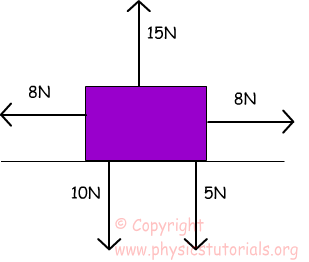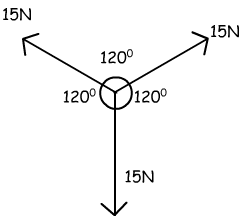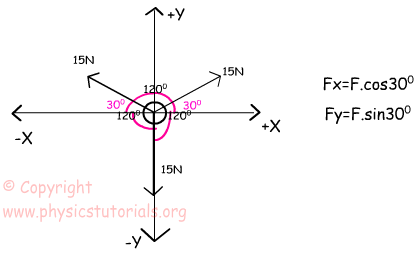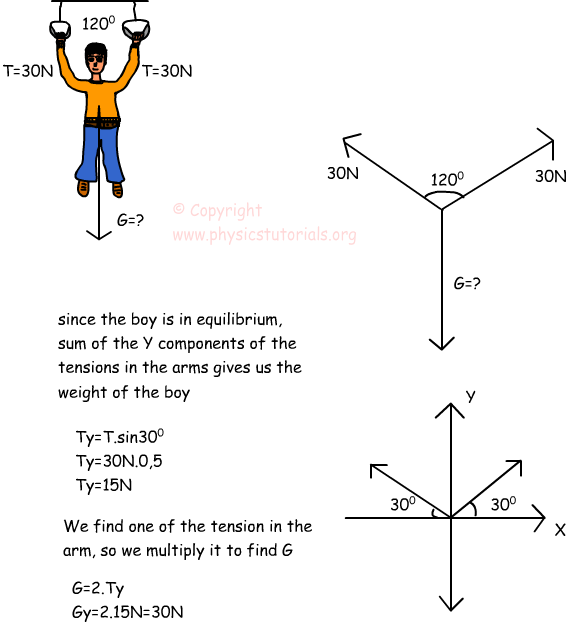# Dynamics Equilibrium with Examples

Equilibrium Conditions: Equilibrium in physics means, forces are in balance. The net force should be zero. In other words, forces acting downward and acting upward, and forces acting right and acting left should be equal in magnitude. Look at theexample given below and try to understand what I say.

In the given picture there are five forces acting on our block. Let us analyze them, whether this block is in equilibrium or not. Forces acting downward are; 10N+5N=15N Force acting on upward is; 15N. Since the directions are opposite and magnitudes are equal these forces balance each other. Thus, we can say that, our block is in equilibrium in +y, -y direction. Now, look at the forces acting right and left. As you see, they are also equal in magnitude and opposite in direction. As a result looking at the fours directions show us to say our block is in equilibrium, all forces are in balance, in other words, net force is zero acting on the object. Look at the other example given below.This system is also in equilibrium. Let’s see how it can be possible.Using the trigonometry, we find the components of force vector. As you can see from the given picture, the X and Y component of the vectors have same magnitude since they have same angle to the horizontal. However, their directions are opposite. Thus, the net force acting on the object becomes zero which is the condition for equilibrium.

Example If the boy is in equilibrium, find the G from the given data in picture. (sin30 º =0, 5, cos30º=?3/2)Dynamics Exams

Related